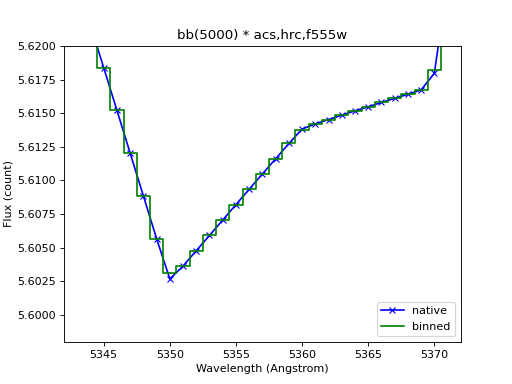# Observation¶

Observation is a special type of Source Spectrum, where the source is convolved with a Bandpass; i.e., a photon has already passed through the telescope optics. It is usually the end-point of a chain of spectral manipulation. Unlike a regular source spectrum, there is only one way to create an observation; i.e., by passing in source and bandpass objects into its constructor. Operations that do not make sense in the context of an observation (e.g., redshifting, tapering, addition, and subtraction) are disabled.

An observation also understands detector binning. By default, the bins are assumed to be the same as the waveset of the input bandpass. However, this is not always true, particularly for “obsmode” in stsynphot. In those cases, the bandpass has an extra binset attribute (wavelength values for bin centers) that must be passed into an observation’s constructor, as shown below:

>>> import stsynphot as stsyn
>>> from astropy import units as u
>>> from synphot import Observation, SourceSpectrum
>>> from synphot.models import GaussianFlux1D
>>> sp = SourceSpectrum(GaussianFlux1D, amplitude=1*u.Jy,
...                     mean=6000*u.AA, fwhm=100*u.AA)
>>> bp = stsyn.band('acs,hrc,f555w')
>>> obs = Observation(sp, bp, binset=bp.binset)


It has these main general components:

• spectrum, the input source spectrum

• bandpass, the input bandpass

• model, the underlying Astropy composite model

• waveset, the wavelength set for “native” sampling

• waverange, the range (inclusive) covered by waveset

• meta, metadata associated with the observation

• warnings, special metadata to highlight any warning

It also has these components related to binning:

• binset, center of the wavelength bins

• bin_edges, edges of the wavelength bins

• binflux, binned flux computed by integrating the “native” flux over the width of each bin

To evaluate its flux at a given wavelength (not binned), use its __call__() method as you would with any Astropy model (except that the method also takes additional keywords like flux_unit for flux conversion). To get binned flux values, use sample_binned(), where you must provide the exact bin center(s):

>>> obs(6000.5)  # Native (not binned) sampling; Angstrom
<Quantity 0.03080553 PHOTLAM>
>>> obs.sample_binned([6000, 6001])  # Binned flux in given centers
<Quantity [0.03084873, 0.0307453 ] PHOTLAM>


To calculate bin properties such as covered wavelength or pixel ranges, you can use its binned_waverange() and binned_pixelrange() as follows:

>>> # Wavelength range covered by 10 pixels centered at 5500 Angstrom
>>> obs.binned_waverange(5500, 10)
<Quantity [5495.5, 5505.5] Angstrom>
>>> # Pixel range covered by above wavelength range
>>> obs.binned_pixelrange([5495.5, 5505.5])
10


In addition, it has unique properties such as Effective Stimulus and Effective Wavelength, which can be calculated in a way that is consistent with ASTROLIB PYSYNPHOT:

>>> # Effective stimulus in FLAM
>>> obs.effstim(flux_unit='flam')
<Quantity 4.12569567e-14 FLAM>
>>> # Effective wavelength for binned sampling in FLAM
>>> obs.effective_wavelength()
<Quantity 5991.99794956 Angstrom>
>>> # Repeat for "native" sampling
>>> obs.effective_wavelength(binned=False)
<Quantity 5991.99798818 Angstrom>


countrate() is probably the most often used method for an observation. It computes the total counts (a special case of effective stimulus) of a source spectrum, integrated over the bandpass with some binning. By default, it uses binset, which should be defined such that one wavelength bin corresponds to one detector pixel:

>>> area = 45238.93416  # HST, in cm^2
>>> obs.countrate(area)
<Quantity 137190.19332899 ct / s>


An observation can be converted to a regular source spectrum containing only the wavelength set and sampled flux (binned by default) by using its as_spectrum() method. This is useful when you wish to access functionalities that are not directly available to an observation (e.g., tapering or saving to a file).

To accurately represent binned flux visually, especially in a unit like count that is very sensitive to bin size, it is recommended to plot the data as a histogram using binset as mid-points, as shown below:

import os
import matplotlib.pyplot as plt
from astropy.utils.data import get_pkg_data_filename
from synphot import Observation, SourceSpectrum, SpectralElement, units
from synphot.models import BlackBodyNorm1D
# Construct blackbody source
sp = SourceSpectrum(BlackBodyNorm1D, temperature=5000)
# Simulate an instrument bandpass with custom binning
bp = SpectralElement.from_file(get_pkg_data_filename(
os.path.join('data', 'hst_acs_hrc_f555w.fits'),
package='synphot.tests'))
binset = range(1000, 11001)
# Build the observation and get binned flux in count
obs = Observation(sp, bp, binset=binset)
area = 45238.93416 * units.AREA  # HST
binflux = obs.sample_binned(flux_unit='count', area=area)
# Sample the "native" flux for comparison
flux = obs(obs.binset, flux_unit='count', area=area)
# Plot with zoom to see native vs binned
plt.plot(obs.binset, flux, 'bx-', label='native')
plt.plot(obs.binset, binflux, 'g-', drawstyle='steps-mid', label='binned')
plt.xlim(5342, 5372)
plt.ylim(5.598, 5.62)
plt.xlabel('Wavelength (Angstrom)')
plt.ylabel('Flux (count)')
plt.title('bb(5000) * acs,hrc,f555w')
plt.legend(loc='lower right', numpoints=1)## specutils¶

A specutils.Spectrum1D object can be passed directly into Observation as a source spectrum. For example:

>>> from specutils import Spectrum1D
>>> spec = Spectrum1D(spectral_axis=[499, 500, 600, 601]*u.nm,
...                   flux=[0, 0.1, 0.8, 0]*u.nJy)
>>> obs = Observation(spec, bp, binset=binset)
>>> obs.effstim(u.ABmag)
<Magnitude 32.70048821 mag(AB)>